Revue Roumaine des Sciences Techniques - Mécanique Appliquée, 42, No. 1-2, pp. 27-57 (1997)

Mayeul Arminjon

Laboratoire "Sols, Solides, Structures",

Institut de Mécanique de Grenoble, France.

Warning: Depending on the navigator, the characters (Times) g, used for the gravity acceleration vector, and (Arial) g, used for the spatial metric, may appear in the same font. Also, the square  (d’Alembert operator) may not appear at all. I have a few offprints. M.A. (mailto:arminjon@hmg.inpg.fr)

Abstract- The construction of this theory starts with Newtonian space-time and a tentative interpretation of gravity as Archimedes' thrust exerted on matter at the scale of elementary particles by an imagined perfect fluid or ether. This leads to express the gravity acceleration by a simple formula in which the "ether pressure" pe plays the role of the Newtonian potential. The instantaneous propagation of Newtonian gravity is obtained with an incompressible ether, giving a field equation for pe. For a compressible ether, this equation holds in the static case and results in a non-linear influence of the mass distribution. The extension of the field equation to non-static situations follows the lines of acoustics and leads to gravitational (pressure) waves. To account for metric effects, first the modern version of the Lorentz-Poincaré interpretation of special relativity is summarized. Then Einstein's equivalence principle (EP) is seen as a correspondence between the metric effects of gravity and those of uniform motion with respect to the ether : a gravitational contraction (dilation) of space (time) standards is assumed, and it implies geodesic motion for test particles in a static field. The same field equation is now expressed in terms of the physical space and time metrics in the frame of ether; since both metrics depend on pe due to the EP, it becomes non-linear in pe. In the spherical static situation, Schwarzschild's exterior metric is predicted, but the interior metric differs from GR, though it agrees with the EP. Since pressure produces gravitation also in the proposed theory, no equilibrium is possible for very massive objects. But the gravitational collapse in free fall does not lead to any singularity. Moreover, gravitational waves, and even shock ones, can exist in vacuo also with spherical symmetry.

Keywords: Gravitation, Ether, Relativity, Relativistic Dynamics, Gravitational Collapse.

Postal  address: Laboratoire "Sols, Solides, Structures", E.N.S.H.M.G., BP 53,  38041 Grenoble cedex 9,  France.

1. INTRODUCTION

Of the reasons that may justify the search for alternatives to general relativity (GR), some are comparatively recent : the complete implosion into a point singularity, which is predicted for very massive objects and which could not be detected in the case of spherical symmetry; the isolation of gravitation with respect to the theories of other interactions (this expressed earlier in the problem of unifying gravitation with electromagnetism, and more recently in the difficulties with quantum gravity); and, finally, the problems of interpretation which are due to the need for a non-covariant coordinate condition : Papapetrou  already suggested that different coordinate conditions might lead to different post-Newtonian equations of motion, and Fock  observed (at § 96) that indeed, the coordinates used by Einstein and Infeld differed from harmonic coordinates, used by Fock and Papapetrou, only by second-order (O(1/c4)) terms which play no role in the first corrections to Newton's theory; today the post-Newtonian calculations are very generally formulated in terms of harmonic coordinates [3, 4]. According to Logunov et al. , one has to modify GR into a "relativistic theory of gravitation" such that, essentially, Fock's harmonic condition is organically contained in the new theory, for otherwise GR would not furnish unique predictions. Thus the general covariance is not a sine qua non condition; in this paper, a theory with a privileged frame is investigated. In the writer's opinion, the most fundamental question that remains unsolved by GR was already posed to Newton's theory : how can interactions propagate through empty space ? Why not try to give physical properties to empty space, in such a way that the propagation of gravity as well as the existence of global inertial frames (i.e. as they appear in Newtonian theory) become understandable?  The existence of the zero-point fluctuations makes it necessary to admit that vacuum indeed has physical properties [6,7]. A comparison of Winterberg's ether theory of gravitation  with the one proposed here, which will be designated by ETG for short, is deferred to the Conclusion for convenience.

Already within the Newtonian concept of absolute space and time, gravity can be tentatively interpreted as Archimedes's thrust, due to the pressure of an hypothetical, perfect fluid or ether, surrounding all elementary particles of matter : the condition of validity is that all particles should have the same mass density - or at least that their average density depends only on the pressure in the fluid [8a]. It turns out that Newtonian gravity (NG) was first interpreted in this way a long time ago by Euler . Under the condition that NG must be recovered for an incompressible fluid, a (scalar) field equation is obtained for the ether pressure; after a compressibility is introduced, this "ETG" gives qualitatively correct modifications to NG : a finite wave speed and an advance in the perihelion of planetary orbits which is proportional to the prediction of GR [8a]. To go further, it is necessary to have a description of the relativistic effects. The first step is to realize that the old Lorentz-Poincaré ether interpretation of special relativity can be updated with the same logical thrift and consistency that have made Einstein's theory so convincing [10,11]. The "new" ether interpretation reconciles Lorentz's original view of a true contraction in the absolute motion with respect to the ether, with the reciprocity of the Lorentz transformation in the version found by Einstein; it will be once more summarized in this paper. The possibility of this interpretation and its great interest were recognized by Einstein  himself (see Builder [10a]). Hereafter, the transition is made to the gravitational situation in which the ether pressure (or equivalently the ether density, since the ether postulated here is a barotropic perfect fluid) varies from one point to another - which is the cause of gravity in the proposed ETG. Special relativity holds then only locally, i.e. in a domain where the ether density may be considered uniform. The true Lorentz space-contraction and time-dilation with motion lead naturally to a new formulation of Einstein's equivalence principle, within the ETG : gravitational space-contraction and time-dilation are postulated; with this assumption and when the proposed equation for the field of ether pressure is reinterpreted as relative to the physical, distorted space and time standards, Schwarzschild's exterior space-time metric is exactly recovered in the static situation with spherical symmetry [8b]. Moreover, for any static gravitation field, the geodesic characterization of the motion of free mass points follows from this assumption and Newton's second law (with the momentum including the velocity-dependent mass and all being measured with the local clock and measuring rod), independently of the field equation.

In this paper, a synthetic presentation of this scalar theory of gravitation is given. Several new results are reported : a discussion of the Newtonian limit; an extension to light-like particles of the proof that Newton's second law (interpreted as above) implies geodesic trajectories; a brief study of gravitational collapse and gravitational waves with spherical symmetry. For these two last problems, the situation in this theory differs strongly from that in GR.

2. THE ETHER THEORY OF GRAVITATION WITHIN NEWTONIAN MECHANICS

Following physicists of the past like Euler, Helmholtz and Kelvin, it is found that only a perfect fluid, i.e. without viscosity, could fill the space left by matter without braking any motion : this is well-known in fluid mechanics as d'Alembert's paradox. Such a fluid acts only by its pressure pe; it would exert over a material domain  W , the force

F = ò¶W  -pe n dS  =  -  òW  grad pe dV    (1)

(W is the boundary of W, n is the outward normal, S and V are the surface and volume measures). We want that F is the gravitation force :

F =   òW  r g dV   ,         (2)

with g the gravity acceleration and r the mass density. If we think of W as being a macroscopic domain where g may be considered uniform (and for the real gravity this is true even with rather large domains), this equality cannot hold, since g in (2) and grad pe in (1) should not depend on the kind and state of matter in W, while r of course does. However, the macroscopic matter is made of particles which are already subjected to the gravitation, hence the fluid pressure would have to act only on a small part of the macroscopic volume W : the union of the volumes wi (i=1,N(W)) occupied by the constitutive particles. In other words, the fluid would fill the place left by the particles. Let ri be the mass density of particle (i). In order that (1) and (2) coincide when W=wi and r=ri, it is necessary that ri be independent of the particle (i), and then the pressure force (1) would also be a mass force (2) for a macroscopic domain W, provided that  grad pe also be uniform in W :

F = - grad pe S  V(wi ) = g S mi =    g S ri V(wi ) .          (3)

Thus, we come to the conclusion that one should have :

g = - grad pe /rp     ,                                                          (4)

with rp the assumed common density of the particles - which could still depend on the pressure pe in the thought ether. Now the identity between inertial and passive gravitational mass does not seem to be known with the same precision for elementary particles than for macroscopic matter. The density in the particles might thus be allowed to vary from one particle to another, so we take for rp the average mass density in the different particles of a macroscopic domain W : rp= S riV(wi)/ S V(wi). But in order that g in (4) does not depend on the kind of present matter, rp must be a function of the state parameters of the ether alone. Since the ether is assumed to be perfectly continuous at any scale, no temperature and no entropy can be defined for it, so the state parameters reduce to the pressure pe and the "mass" density re which are related together by the barotropic state equation ; hence rp must depend only on pe. This seems to be possible only if the particles of matter are themselves ether : from the attempt to describe gravity as a pressure force in a fluid ether, we are lead to the assumption of a constitutive ether, which was a priori set by Romani .

Assuming therefore that re = rp , we obtain the gravity acceleration as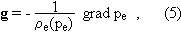but this equation may be taken as a phenomenological equation for g (alternative to the Newtonian equation g = grad U ; note that in Newtonian theory, the potential U, as here the pressure pe of the imagined fluid, manifests itself only through g). The foregoing microscopic considerations are then a heuristic justification for Eq. (5). We note that pe and re should be the macroscopic pressure and density in the thought ether, since the gravitation varies only over macroscopic distances and due to the effect of macroscopic bodies. This remark is important in the context of a possible unification of the four known physical interactions within a theory of this barotropic ether : the other interactions, which vary over shorter distances, would then have to be all contained in the microscopic fluctuations of ether pressure and velocity. In fact, Romani  described a way in which this may be done, in assuming that all known particles would be vortex tori or complexes of such flows; he proposed to explain the appearance of quantum numbers from the requirement that the helicoidal trajectories on the torus should be closed. Yet his work, while very interesting, suffers in some places from a lack of rigour, and contains no field equation. Furthermore, gravity was the least developed part of his work and if he attributed this force to a gradient of ether density in the barotropic ether [13, vol. 1], he stated that the deviation of light rays passing near the Sun should be due to an increase of this density towards the Sun [13, vol. 2] - whereas it follows immediately from our Eq. (5) that pe and re decrease towards the gravitational attraction. In the present work, our aim is to deduce from the assumption of the barotropic constitutive ether a phenomenological theory for gravity, in a classical framework, i.e. without trying to obtain a quantized gravity, and also without any claim of describing the other interactions. Inasmuch as a theory of gravity has to be compared with NG and GR which are also phenomenological theories, we thus do not care about the fact that, of course, quantum mechanics would scarcely allow to define a mass density for an elementary particle, and would not allow to use classical mechanics at this level : within the Newtonian space-time, the theory describes gravity by a potential (Eq. (17a) below) which might be used as a phenomenological description of this interaction, also at the quantum level, in non-relativistic quantum mechanics. Also, once modified to account for "relativistic" effects, the investigated theory, just like GR, will finally lead to a curved space-time, the curvature being bound to the distribution of matter; this is in turn adapted to describe gravity in relativistic quantum mechanics.

In this phenomenological view, the next step is to recover Newtonian gravity. This is a straightforward task, for NG propagates with infinite velocity and thus must correspond to the incompressible case; with a uniform re , we get from Eq. (5) by using Poisson's equation (div g = -4 p G r with G Newton's gravitation constant) :

Dpe = 4p G r re    .                                           (6)

With a uniform re , Eqs. (5) and (6) give exactly the same description of the field g as the usual equations DU = -4 p G r and g = grad U. The point is that (5) and (6), together with the barotropic equation re = re(pe) (of which the incompressible case is a degenerate one), make sense as well if the ether is compressible (i.e. when re is an increasing function of pe). In that case, however, we have a new, non-linear field equation for gravity : the fields pe and g depend non-linearly of the matter distribution r, even if the pe-re relationship is linear. The static case with spherical symmetry has been investigated analytically : one obtains a complete solution that tends towards the Newtonian one as the compressibility tends towards zero, and the exterior solution gives an advance in the perihelion of elliptic orbits which is proportional to the prediction of GR [8a]; in ref. [8a], an extension of Newtonian mechanics to fluid inertial frames is also proposed, consistently with the assumption that the frame bound to the macroscopic motion of the fluid ether (the "macro-ether") defines an inertial frame. In the case where the relationship is assumed to be pe=rec2 with c the velocity of light (as we will be lead to, Sect. 4), Eq. (6) writes

Dpe = (4p G/c2) r pe                                           (6bis)

which is formally identical to a relativistic equation proposed for static situations by Einstein  before he finally came to GR; yet in Einstein's equation the field was of course not pe but instead the velocity of light w, as expressed in terms of a "universal" time coordinate [this circumstance was not known to the writer for the work ; the writer learned this in the papers by Soós , who presents a systematic analysis of Kepler's problem in Einstein's successive theories of gravitation].

A motion of massive bodies causes a disturbance in the gravitation field, i.e. in the field of ether pressure pe; but with a compressible ether, this disturbance in the field pe cannot propagate instantaneously and instead should propagate as a pressure wave. A non-static situation is thus obtained. On assuming that : (i) the ether is conserved; (ii) the disturbed motion of the ether, i.e. its motion with respect to the "macro-ether" which defines the inertial frame, obeys Newton's second law; (iii) the disturbance in the fields (pe1 for ether pressure and ve1 for  ether velocity) is small, the latter being assessed with respect to the "sound" velocity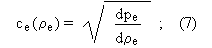and (iv) the undisturbed fields pe0 and re0 of a local decomposition

pe=pe0 + pe1 ,  pe1 << pe0  and  pe0/t << pe1/t     (7bis)

obey Eq. (6), it has been proved in [8a] that the general, non-static situation is governed by the equation :

Dpe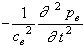= 4p G r re    .  (8)

Equation (8) clearly admits pressure waves, i.e. gravitational waves propagating at the velocity ce(re).

The "macro-ether" is a primary concept in the proposed theory. With respect to an arbitrary reference frame R, the velocity ve0 of the macro-ether is related to that of the microscopic ether (that which is thought to be concerned with particles and shorter-range fields) by an averaging over domains W such that the ether pressure is uniform in W (but varies over larger scales).

3. SPECIAL RELATIVITY AND ETHER THEORY

It is well-known that Lorentz and Poincaré, whose analyses were dependent on the assumption of the rigid luminiferous ether, derived some basic results (formulae) of special relativity (SR) before Einstein who's starting point was the relativity principle. Our point here is not to raise a discussion on the extent to which Lorentz and Poincaré did open SR : it is only to briefly recall why the whole of SR, in its usual formulation due to Einstein, can be consistently derived from the above ether assumption; this has been established by several physicists among which Builder  and Prokhovnik . The crucial step is to recover the usual Lorentz transformation from the mere assumption (Fitzgerald and Lorentz) of an absolute contraction of all material objects when carried over from the rest frame E of the ether to a frame Eu that moves with uniform and constant velocity u with respect to E. The contraction occurs on lines parallel to u, but not on perpendicular lines. Its ratio b(u) depends only on the modulus u=|u| (this results from the assumed isotropy of electromagnetic interaction in the frame E), in a way that does not need to be assumed in advance [8b]. Let l be the length of a rod AB, when it was at rest in E, and l' its length, evaluated from E, when this same rod is now at rest in Eu and makes, for E, the angle q with u. The assumed contraction implies that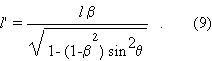Consider AB (with a mirror at A perpendicular to AB and the like at B) as a "light clock", its time unit being the interval during which the light goes from A to B back and forth. To calculate the time unit Dtu(q), for E, of this light clock as it is now in Eu, we need only space and time measurements in E, so we can write if a light wave front going from A to B intersects the rod at M (and the point O being bound to E):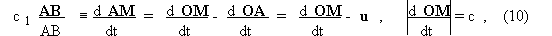whence c1 =- u cos q and similarly c2 =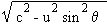+ u cos q in the BA direction. Therefore,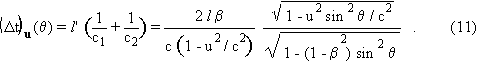The "negative" Michelson-Morley experiment means exactly that this period does not depend on angle q and thus is a constant in the moving frame Eu. From (11), we see that this is true if and only if one has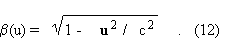The negative Michelson experiment means that light clocks are correct clocks, and this implies the Lorentz contraction. Moreover, the time unit of Eu is "dilated" : (Dt)u = Dt/b. Prokhovnik shows precisely that these results, together with the standard synchronization of clocks, imply the kinematics of SR in Einstein's form, i.e. imply first the constancy of c (as this is measured with clocks and rods of any frame in uniform motion Eu) and then the usual Lorentz transformation . The difference with the usual version is in the perfectly clear interpretation of the space contraction and time dilation as absolute effects. The relativity of simultaneity is interpreted as an artefact due to the necessity of synchronizing clocks with light signals and to the anisotropic "true" propagation of light, Eq. (10), in a frame that moves with respect to the propagating medium E. The true simultaneity is defined with the time of E, and could take an operational significance if the velocity with respect to E could be known.

Then, the dynamics of SR follow essentially from its kinematics and from the requirement to save Newton's second law in E as well as at least a weak form of the principle of inertia (WPI) - namely that the momentum conservation for an isolated system of mass points pass from E to Eu [8b,11]. One realizes first that this can be true only if the inertial mass depends on the relative velocity, m=m(v). On assuming that the m(v) dependence is the same in E and in Eu , the WPI leads to the expression of m(v) and the kinetic energy T in SR :

m(v) = m(v=0)/b(v) ,   T = (m(v)-m(0)) c2    .         (13)

Einstein's form of Newton's second law is thus obtained in E :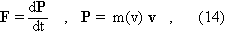and the requirement that it is Lorentz-covariant, i.e. holds true when passing from E to Eu , determines the transformation of F. The momentum conservation can be postulated also in the case of non-conserved rest masses (i.e. creation/annihilation processes), and this extension also passes from E to Eu if and only if the total mass is conserved :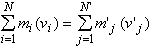.             (15)

Together with Eq. (13)2, this gives the classical argument of SR for attributing to any particle the "rest energy" m(0)c2 and identifying mass and energy, up to the factor c2.

4. EQUIVALENCE PRINCIPLE AND ETHER THEORY

As shown by Fock  and Synge , Einstein's equivalence principle (EP) between effects of a gravitational field and inertial effects has to be used with some care; in particular, it is only valid in the infinitesimal, i.e. for uniform fields. Yet in a relativistic theory, the unifor-mity must be in space-time (i.e. the infinitesimal domain to consider is in space-time), thus the local equivalence should already apply with a uniform and non-accelerated motion. Hence a such motion should have "inertial" or pseudo-gravitational effects. This is readily verified, for in GR gravity manifests itself by effects on clocks and rods, and effects of this kind are indeed predicted by SR for non-accelerated motion; and again because the equivalence is for uniform fields, the metric effects of non-accelerated motion should be the essential ones when looking for the metric effects of gravity. This is apparent in Einstein's rotating disc. In this example, the metric effects are indeed assumed to depend on the local velocity, v = rw (w is the constant spin rate of the disc with respect to the inertial reference frame). Moreover, the pseudo-gravitational (acceleration) field has intensity a = rw2 er = grad (v2/2); since the radial distance r is not fixed, the metric effects are bound in no way to the local intensity of the field, but instead to its potential (the same conclusion is drawn if one considers an accelerated translation). Thus, an analysis based on EP indicates unambiguously that the effects of gravitation on the space-time metric : (i) are bound to the gravity potential rather than to the field intensity, and (ii) should be deduced from the effects of non-accelerated motion. In the orthodox relativistic view, the second conclusion is difficult to accept, because no inertial frame is privileged there and the metric effects of non-accelerated motion are hence a kind of "parallax in space-time" rather than absolute ones, whereas the presence of a gravitational field is surely something "absolute", that cannot be removed by changing the reference frame - except, and for one part only, in the infinitesimal. The EP together with Einstein's lift and disc are often used in GR to introduce Einstein's idea that gravitation is (nothing else than) a curved space-time metric with (1,3) signature, the geodesic lines of which are the trajectories of free test particles. However, Einstein's field equations cannot be obtained directly as a modification of Newton's accounting for EP, and this might be related to the above-mentioned difficulty with the absolute effects of uniform motion.

In a theory based on ether, there is no logical difficulty in assigning absolute effects to uniform motion with respect to the ether. Furthermore, there is in the proposed ETG a basis for the EP when this is seen as a relationship between the metric effects of motion (depending only on the velocity, i.e. on the locally tangent uniform motion) and those of gravitation. Gravitation is for us a variation in the ether density re , and a variation in the apparent ether density indeed occurs in uniform motion, due to the Lorentz contraction : for an observer having a constant velocity u with respect to E, a given volume dV0 of ether has a greater volume dV=dV0/b(u), because his measuring rod is contracted in the ratio b in the direction u. The "mass" or rather the amount of ether is unchanged, for the mass increase with velocity concerns only material particles : the "mass" of the macro-ether is not a mass in the mechanical sense [8a]. Thus the apparent ether density is lowered, reu = re.b(u). This way of reasoning assumes that the moving observer can use the "true" simultaneity (of the frame E), which is true if he knows his velocity u; with the simultaneity defined from clock synchronization in the frame Eu, the apparent ether density would be r'eu = re/b(u) since dV'=dV0.b(u) as deduced from standard Lorentz transformation. In any case, the metric effects of uniform motion are given by the ratio of an apparent ether density to that of the reference ether density, b(u)=reu/re = re/r'eu <1. It is therefore natural to postulate that :

(A) In a gravitation field, material objects are contracted, only in the direction of the field g=-grad pe/re , in the ratio

bg = re / re¥ < 1 ,                                   (16)

where re¥ is the ether density at a point where no gravity is present, and the clock periods are dilated in the same ratio.

This statement is made for objects and clocks bound with E; if this is not so, one has to combine the metric effects due to motion and gravitation. Due to the space-contraction of measuring rods in the direction g, the physical space metric g in the frame E becomes a Riemannian one. The contraction occurs with respect to an abstract Euclidean metric, which we assume that the macro-ether may be equipped with, as a 3-D manifold M : we assume that M is diffeomorphic to R3 ; let us precise that M is the same manifold at any time, since the body "macro-ether" (Sect. 2) is followed from its own rest frame. However, an infinity of different flat metrics may be defined on a such manifold . It is thus more exact to formulate the above assumption in stating : that space metric g0 (in the frame E) which is (uniquely) deduced from the physical one g by the dilation of length standards in the ratio re¥/re >1 in the direction g , is a flat metric . Therefore, the value re¥ does not need to be reached : it is the unique value for which the assumption holds. There are three further arguments for assumption (A) :

(i) according to the ETG, the gravity acceleration g  derives from the potential :

U = -ce2 Log(re/re¥),                                                                 (17a)

with = ce(re) (this is deduced from Eq. (5) after linearization of the pe - re relationship around re¥ and holds thus either for a weak field or if the pe - re relationship is linear); for a weak field:

U » ce2 (re¥ - re)/ re¥ ,        1 - U/ce2 » re / re¥                          (17b)

Furthermore, in the theory of the fluid constitutive ether, the material particles are local flows in ether (vortex tori, or complexes of such flows) and therefore their speed is limited by the local "sound" velocity ce . Since SR gives the other limit c, one must have ce º c, which implies that the pe-re relationship is in fact linear, pe = c2 re . Now it is well-known that the EP implies that in a weak field any clock is slowed down in the ratio b = 1 - U/c2 (this follows immediately from Einstein's analysis of the disc or lift, cf. supra), hence the EP together with Eq. (17) give Eq. (16) for weak fields.

(ii) It is easy to check that if one states assumption (A) without giving the ratio bg by Eq. (16), this latter (possibly combined with the a(t) factor, see above) is equivalent to say that, as evaluated with respect to the Euclidean metric g0, the ether density is uniform (in space). Since this metric is by hypothese bound to the ether, i.e. makes the ether a rigid body, it is only natural that it renders the ether density uniform and thus the unknown ratio bg must be given by Eq. (16). This result also confirms, if there could be any doubt, that in the modification of the ETG accounting for SR and EP, the equations will have to be understood as relative to the physical metric g instead of the abstract metric g0 , for the ETG assumes an ether compressibility K¹0 (and now Kº1/c2).

(iii) Let us investigate whether the ETG leaves the possibility of a Newton law in the frame E, in the presence of gravitation and Riemannian space-time (as already seen, the space and time standards must be the local, physical ones). The time-derivative of a vector (the momentum) may be consistently defined as a vector in the case of the Riemannian metric g on the manifold M, if g is time-independent [8b]. If P (e.g. the momentum of a mass point) is a vector attached to a moving point whose position x in M depends on the arbitrary time-coordinate t, one defines then its derivative (relative to this coordinate t) by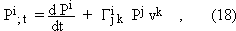with vk = dxk/dt and the G's being the Christoffel symbols associated with the metric g=(gij) in the considered coordinate system (xk); this defines a true vector (the components (18) are contravariant with respect to any transformation of space coordinates). Thus an exact Newton law can be defined if re and hence g do not depend on time. The expression of the momentum is known from SR, P=m(u)u, the velocity u and its modulus u being defined with clocks and rods of the momentarily coincident point of M : uk=dxk/dtx=vk dt/dtx , tx being the local time defined by assumption (A) : if t=tx0 is the proper time of a fixed point x0ÎM, one gets in general from Eq. (16) :

dt/dtx  = dtx0/dtx = re(x0,t)/re(x,t) = re0/re ,     (19)

though in the present case re does not depend on t. The gravitation force involves the gravity acceleration g (Eq. (5) where the gradient is defined with g) and the passive gravitational mass mp. According to the EP, in its weak form already true in Newtonian theory, mp equals the inertial mass, here m(u). Thus our modified Newton law writes :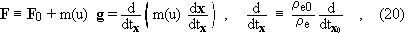where F0 is the non-gravitational force and the time derivative of the momentum is calculated with Eq. (18); equation (20) is invariant under the change of the reference point bound with ether, x0. Now we have the following result [8b] :

(G) if the gravitation field is constant in the frame E, then the solution trajectories of the modified Newton law with purely gravitational force, Eq. (20) with F0=0, are geodesic lines of the space-time metric deduced by assumption (A); the line element of this metric g writes  in the frame E  :

ds2 = c2 dt2 = (re/re0)2 (dx0)2 - dl2  ,           (21)

where t is the proper time of the test particle, x0=ct (t=tx0) and dl2 is the space line element calculated with the space metric g in the frame E :

dl2 = u2 dtx2  ,   u2 = g(u,u) = gij ui uj .         (22)

Thus, the modified Newton law (20), which is not even Lorentz-covariant, implies the geodesic characterization of motion which is generally covariant. We assume here, by induction from the result (G), that free test particles also follow the geodesic lines of g if re and g are time-dependent, in which case we have not defined a Newton law. However, if the time variation of re is small in such a way that the corresponding terms are negligible in the equation of space-time geodesics (or more generally in the expression of the four-acceleration of any test particle, "free or not"), then an approximate Newton law (20) is obtained by still using the definition (18) for the derivative of the momentum. Note that, due to Eq. (21), the components g0i (i=1,3) of g are always nil in the frame E. In particular, a constant (time-independent) re corresponds to what is called a static field in GR.

5. FIELD EQUATION, NEWTONIAN LIMIT AND INERTIAL FRAMES

The result (G) does not depend on the field equation. To adapt this latter to the situation where the metric modifications due to motion and gravitation are accounted, we first note that, in the static case, the whole of the arguments in Sect. 2 may be repeated to obtain Eqs. (5) and (6), but this time with the Riemannian space metric g (the requirement that Newtonian theory must be recovered for an incompressible ether remains as it stands since, from Eq. (16), the Euclidean metric is regained simultaneously with Poisson's equation as the compressibility tends towards zero). In the non-static case, the reasonment, detailed in [8a] and based on the assumptions (i) to (iv) in Sect. 2 here, is not so easy to modify in a clear-cut way, in so far as we have no Newton law any more. However, the same equation (8) should be obtained in terms of the physical space and time metrics in the frame E  :

Dg pe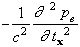= 4p G r re    .  (23)

Indeed, the proposed approach leads unambiguously to this equation in the static case, and Eq.(23) is merely the natural expression of Eq. (8) once it has been recognized that the non-uniform physical space and time metrics are relevant. Thus one is strongly inclined to assume Eq. (23) - and so we do. We emphasize, however, that in (23) the Laplace operator D is defined with the physical space metric g which is curved and whose expression in terms of the Euclidean metric g0 bound to M, depends on the unknown pe through assumption (A); in the same way, the local time tx depends on pe or re (Eq. (19)). Hence the left-hand side of Eq.(23) is not that of d'Alembert's classical wave equation and instead depends non-linearly on pe . It is also worth to note that the operator on the left of Eq. (23), which is linear if g or pe is considered given, but which is here precisely applied to pe , is not even the modified d'Alembert or  operator of GR, which is defined from the Riemannian space-time metric g by

 f = divg (gradg f) = (1/Ö|g|) (/xa)(Ö|g| gab f/xb)  ,  (24)

with g = det (gab). In fact, the Laplace operator in (23) is defined from the same formula by substituting the space metric g for the space-time metric g , and thus with summation on latine indices i and j (from 1 to 3) instead of that one on greek indices a and b (from 0 to 3) in Eq. (24) :

Dgf = divg (gradg f) = (1/|g|) (/xi)( Ö|g| gij f/xj)  .  (25)

Due to this non-linearity, Eq. (23) contains features of classical equations for intense wave propagation (e.g. those of gas dynamics), in particular it contains the possibility of shock waves (Sect. 8). There is another non-linearity in Eq. (23), since its right-hand side already contains a non-linear dependence of pe on r; in a sense, this right-hand side implies that the gravitation field, characterized by pe or re , is a source to itself. Moreover, the mass-energy equivalence of SR has to be accounted for in the definition of the mass distribution r. Thus r in Eq. (23) is defined to be the density of mass-energy in the frame E. As imposed by the inclusion in r of the energy of light-like particles (divided by c2), the mass of ordinary particles involved in r is not the rest-mass but the velocity-dependent one, which is to say that the Newtonian equality of active and passive gravitational mass is saved. It implies that the mass equivalent of the ordinary, kinetic pressure p in macroscopic bodies is included in r, in other words pressure does contribute to gravity in the ETG, as in GR.

It appears that Eq. (23) has no covariance property, but this is a priori tenable since all quantities are understood in the frame E, which according to the theory is strongly privileged. The next thing to do is thus to show that the Newtonian approximation, which for most of the reliable observations is very accurate and which implies the Galilean invariance, is recovered for weak and slowly varying fields in this modified ETG. In GR, such fields are characterized by the property that one may choose the coordinate system (xa) so that the space-time metric g differs from the Galilean one h (with hab = dab if a=0 and hab = -dab if a=1,2,3) only by evanescent terms : gab = hab + hab and moreover the derivatives h/x0 , with x0=ct, are negligible as compared with the space derivatives h/xi. Similarly, we admit that the field pe has the form

pe = pe¥(1-U/c2),  U/c2 <<1 ,    (26)

and we will neglect the derivatives of the kind pe/x0 with respect to the corresponding space derivatives. Eq. (26) obviously means that the gravitation field in the sense of the ETG (g in Eq. (5)) is weak; moreover, the deviations from the Euclidean space metric g0 and the uniform time t are directly given by the ratio pe/pe¥ (Eq. (16)). If we select a Cartesian coordinate system (xi) on M, i.e. g0ij=dij , it follows thus from Eq. (26) that the Laplace operator D according to g (Eq. (25)) will differ negligibly from that one according to g0, D0 f=2f/xixi, and that the derivatives with respect to the local time tx may be replaced by ones with respect to the time t. If we account further for the assumption of a slowly varying field, we rewrite Eq. (23) in the form

D0 pe = 4pG r re » 4pG r re¥.       (27)

Now with pe = c2 re, the field g = - (grad pe)/re is, by Eq. (26), equivalent to -(grad pe)/re¥ , thus we recover the incompressible case with Euclidean metric, i.e. the Newtonian equation for the gravitation field :

D0 U = - 4pG r  .     (28)

In other words U in Eq. (26) is equivalent to the Newtonian potential. It may be worth to give a simple reason why the spatial derivatives should dominate the x0-derivatives in the Newtonian limit. The Newtonian potential is the convolution product : U=Gr*(1/r) with r the spatial distance, so that:

U/x0 = (1/c) U/t = (1/c)Gr * (1/r)/ t = (1/c)Gr * [(r/t).grad(1/r)] , (29)

and -r/t in (29) is the velocity of the current point y in the set of massive bodies, with respect to the point x where the field is computed. Thus for low velocities one has |r/t| << c for any y and U/x0 is indeed negligible with respect to grad U.

The Newtonian field equation (28) may be written in any reference frame (as far as the space metric is considered Euclidean) and thus does not define the inertial frames. These are obtained from the Galilean invariance of the non-relativistic Newton law; in the ETG, the latter is derived in exactly the same way as in GR, from Einstein's geodesic assumption for weak and slowly varying fields and small velocities. In this derivation, the x0-derivatives of the metric are again neglected. Thus the approximate inertial frames that appear for weak and slowly varying fields are such that the corresponding expression of the metric is close to be static, as is confirmed by the result (G). The basic assumption (A) (the gravitational space contraction in the ratio b of Eq. (16)) relates the physical space metric in the frame E to the abstract Euclidean metric which makes the macro-ether a rigid body. Thus, in stating assumption (A) we force the ether to remain the absolute space also in the modified version of the proposed theory; moreover, the iterative approximation of the proposed ETG confirms that the inertial frames of the first (Newtonian) approximation are in uniform motion with respect to the macro-ether (this iterative scheme is not presented in this paper).

Note that in the static case (re time-independent), Eq. (23) implies that r also does not depend on t in the frame E; since in that case the modified Newton law holds, there can be only one body (any other body would fall, in contradiction with the constancy of r) and this body must be in equilibrium and at rest in the frame E. Thus in the static case there is no relative motion of ether and matter at the macro-scale. There is another case where the proposed ETG leads to this "total dragging" situation. We have postulated a constitutive ether: according to this, material (elementary) particles would be local flows in ether . If one accepts this, then the macroscopic motion of the ether (defining the frame E) must include the motion of matter, since the former motion is a weighting of the motions of the ether "constituting the particles" and of the ether "outside the particles". As realized above, the macro-ether remains the absolute space, hence the particles cannot drag all the surrounding fluid. However, the mean motion of ether is directly influenced by the motion of particles. Since the ether compressibility is assumed to be extremely low (K=1/c2), the "amount" of ether inside the particles contained in a volume W, mepart = òparticles in W re dV, is nearly proportional to the volume they occupy, Vpart = òparticles in W  dV. Hence, at ordinary densities where the particles occupy only a very small volume fraction, the direct (kinematical) influence of the motion of matter on the macroscopic motion of ether is likely to be very small. But consider the case of a body in advanced gravitational collapse, where the material density would be higher than the nuclear density; in that case, it is natural to admit that the particles are "contiguous to each other", i.e. that all ether is involved in material particles : the macro-ether is then automatically comoving with matter (see Sect. 8 for an application).

REFERENCES for Sections 1 to 5

 A. Papapetrou, Proc. Phys. Soc. A64, 57 (1951).

 V. Fock, The theory of space, time and gravitation, Pergamon, Oxford 1964.

 T. Damour and N. Deruelle, Ann. Inst. Henri Poincaré (Phys. Th.) 43 (1), 107 (1985).

 S. Weinberg, Gravitation and cosmology, J. Wiley & Sons, New York 1972.

 A. A. Logunov, Yu. M. Loskutov and M. A. Mestvirishvili, Sov. Phys. Usp. 31 (7), 581 (1988) (Transl. from Uspekhi Fiz. Nauk 155, 369 (1988)).

 D. W. Sciama, in "The philosophy of vacuum" (S. Saunders & H.R. Brown, eds.), Clarendon Press, Oxford 1991, p. 137.

 F. Winterberg, Z. Naturforsch. 43a, 369 (1988).

 M. Arminjon, Rev. Roum. Sci. Techn.- Méc. Appl. 38, (a) 3 and (b) 107 (1993).

 L. Euler, Opera omnia, Ser. 3, i: Commentationes physicae, pp. 3-15 and pp.149-156            (1746).

 G. Builder, Austr. J. Phys. 11, (a) 279 and (b) 457 (1958).

 S. J. Prokhovnik, The Logic of special Relativity, Cambridge University Press, Cambridge 1967.

 A. Einstein, Sidelights on Relativity, Methuen, London 1922.

 L. Romani, Théorie générale de l'univers physique (réduction à la cinématique), Blanchard, Paris 1975 (vol. 1) and Paris 1976 (vol. 2).

 A. Einstein, Ann. d. Phys. 38, 355 (1912).

 E. Soós, Rev. Roum. Sci. Techn.- Méc. Appl. 37, (a) 127 and (b) 245 (1992).

 J. L. Synge, Relativity, the general theory, North-Holland, Amsterdam 1964.

 If any diffeomorphism of M onto R 3, i.e. any everywhere regular coordinate system (xi), is given, one simply sets g0ij(x)=dij for all x; hence, even what is defined as "straight lines" is not fixed, and instead may be deformed very generally from one choice to another.

 However, there is still the possibility that g and g0 are related together by assumption (A) up to a time-dependent factor a(t) only : this will be important in cosmological problems, since it allows a global expansion of the ether relative to the physical space metric. Note also that R3 might be replaced by the sphere S3 (resp. the Lobatchevsky space L3) and hence M would be a space of constant positive (resp. negative) curvature equipped with its natural Riemannian metric g0 .